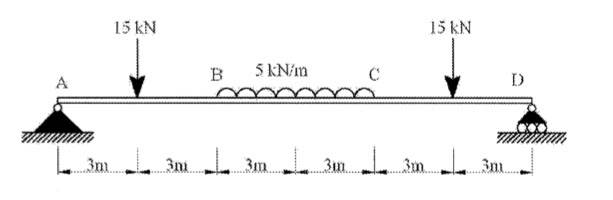# Moments, completely lost

• cd19

## Homework Statement

Calculate the magnitude of the bending moment and shear forces at the centre of the beam shown in the attached figure## The Attempt at a Solution

For the shear forces I think its the sum of the vertical components,
Bending moment = not quite sure.

I would appreciate if someone could give me a few pointers on bending moments and shear forces. Have looked over some textbooks but it really isn't explained well. Appreciate ANY help.

cd19: unfortunately you are dead in the water until a mentor approves your attachment.

I would suggest using a free image host like photobucket.com or imageshack.

Then you will not have to wait for approval, you can just upload your images to photobucket and then copy/paste the image tag into your post.

sorry here it is:Last edited:
Any hints?

You should first determine the vertical end reactions usung the equilibrium equations, or take advantage of the symmetry of the loading to determine those end reactions. Once you have them, the internal shear and the bending moment at the beam's center can be found by applying the equilibrium equations to a free body diagram of the left part of the beam (from its left end to the beam center). The shear at the center is not just the sum of the external vertical forces.

Ok so I have found the vertical end reactions to be:

r1 = 630.25kN
r2 = 8.9167kN

So what i gather is I have to draw a free body diagram exposing sections between the left support and the load. I do this in intervals, summing forces and taking moments about a point in the section.

so what I have attempted is the above from the intervals 0<x<6, 6<x<9, 9<x<12 but how do i solve for x and get a single answer for the shear force and bending moment?

Ok so I have found the vertical end reactions to be:

r1 = 630.25kN
r2 = 8.9167kN
So what i gather is I have to draw a free body diagram exposing sections between the left support and the load. I do this in intervals, summing forces and taking moments about a point in the section.

so what I have attempted is the above from the intervals 0<x<6, 6<x<9, 9<x<12 but how do i solve for x and get a single answer for the shear force and bending moment?
The problem asks for the (internal) shear and bending moment at the center. So just expose the section from the left end to the center. At the center, there wiil be an unknown vertical load (the shear) and an unknown moment (the bending moment). You'll need to solve for these unknowns using sum of F_y = 0 , and sum of moments = 0.

Ok so I see that as everything is perfectly symmetrical, the end reactions at A and D must be 30 Kn respectively.

Also said:
I don't understand why I have to find the external reaction at D.

This is my attempt at the next part:

I have also tried working out the Mbending but i have a feeling my attempt is all wrong, find it very hard to grasp moments, I tend to over complicate.

Ok so I see that as everything is perfectly symmetrical, the end reactions at A and D must be 30 Kn respectively.
yes, correct.
$I don't understand why I have to find the external reaction at D. You already did, but it's good to check your work. This is my attempt at the next part:$Vload+15 = 30 therefore the Vload=15
No, you must sum ALL forces to solve for the unknown shear, V, in your FBD of the left part of the beam. That includes the concentrated load of 15 kN down, the end reaction of 30 kN up, the downward contribution of the distributed load, and V, all summed to 0 to solve for V.
I have also tried working out the Mbending but i have a feeling my attempt is all wrong, find it very hard to grasp moments, I tend to over complicate.
to solve for the bending moment, sum moments about the right end of your FBD. It will be easier to assist you if you show your work.

This Is what I got:

For ''V'' ; 15+15-30+V=0 therefore v = 0 Kn (shear)

Summing the moments about the right end of my FBD i got:

-(30)*(9) + (15)*(6) = 180kNm (Bending Moment)

Is this looking correct?

This Is what I got:

For ''V'' ; 15+15-30+V=0 therefore v = 0 Kn (shear)
Looks Good!
Summing the moments about the right end of my FBD i got:

-(30)*(9) + (15)*(6) = 180kNm (Bending Moment)

Is this looking correct?

I can see you don't like that distributed load, because you keep leaving it out of your FBD! Represent it as a 15 kN load acting at its c.g., then sum moments again. Indicate if the bending moment at the center of the beam is clockwise or counterclockwise.

30*(9) - 15(6) -15(1.5) = bending moment =157.5(Kn) acting clockwise

I would have never of got that distributed load right i.e the perpendicular distance (1.5), I'm terrible at FBDs